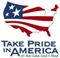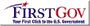Scientific Investigations Report 2006-5188

U.S. GEOLOGICAL SURVEY
Scientific Investigations Report 2006-5188

# Regression Statistics

Regression coefficients and coefficients of determination (R2) for the best-fit regression models for loads of dissolved and total Cd, Pb, and Zn for the 10 studied sites are presented in table 2. Coefficients of determination tended to be somewhat inflated because of the form of the regression equation. Because load is a function of flow (equation 1) a strong relation (high R2) is expected, unless large variations are in concentration. Nevertheless, the relatively high R2 values indicate that, with few exceptions, the models for all constituents successfully simulated the variability in constituent loads at most sites.

Table 2 also shows p-values for coefficients of terms representing time as a variable in the regression equation (coefficient “f”). These coefficients can indicate trends in the load estimations. Because the 5-year interval for these estimates was considered insufficient to yield reliable trend results, they are not discussed here. However, trends for 14-year load estimates are discussed later in the report.U.S. Department of the Interior | U.S. Geological Survey